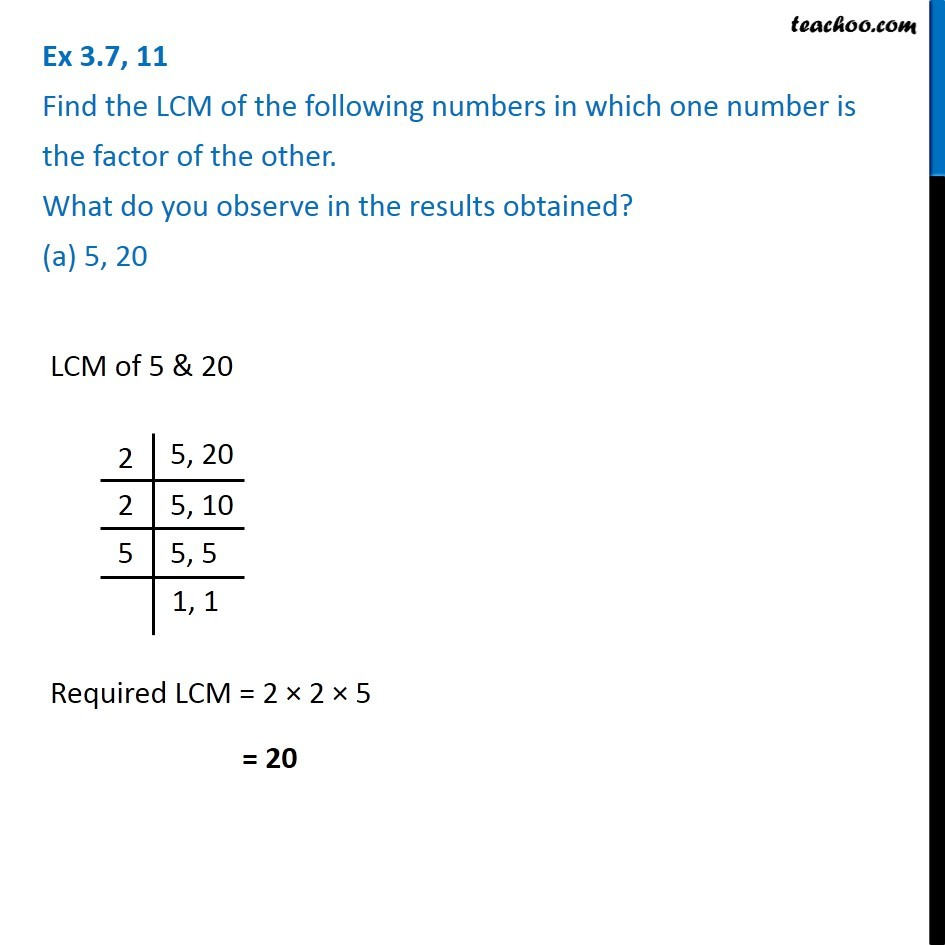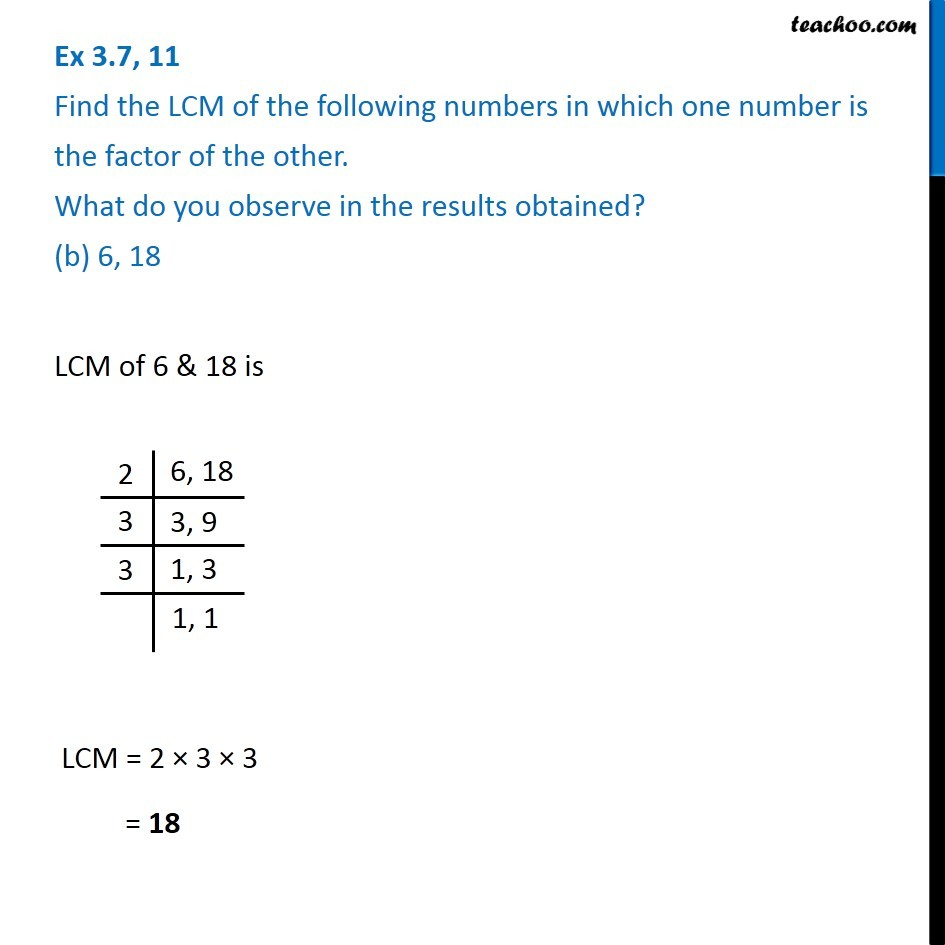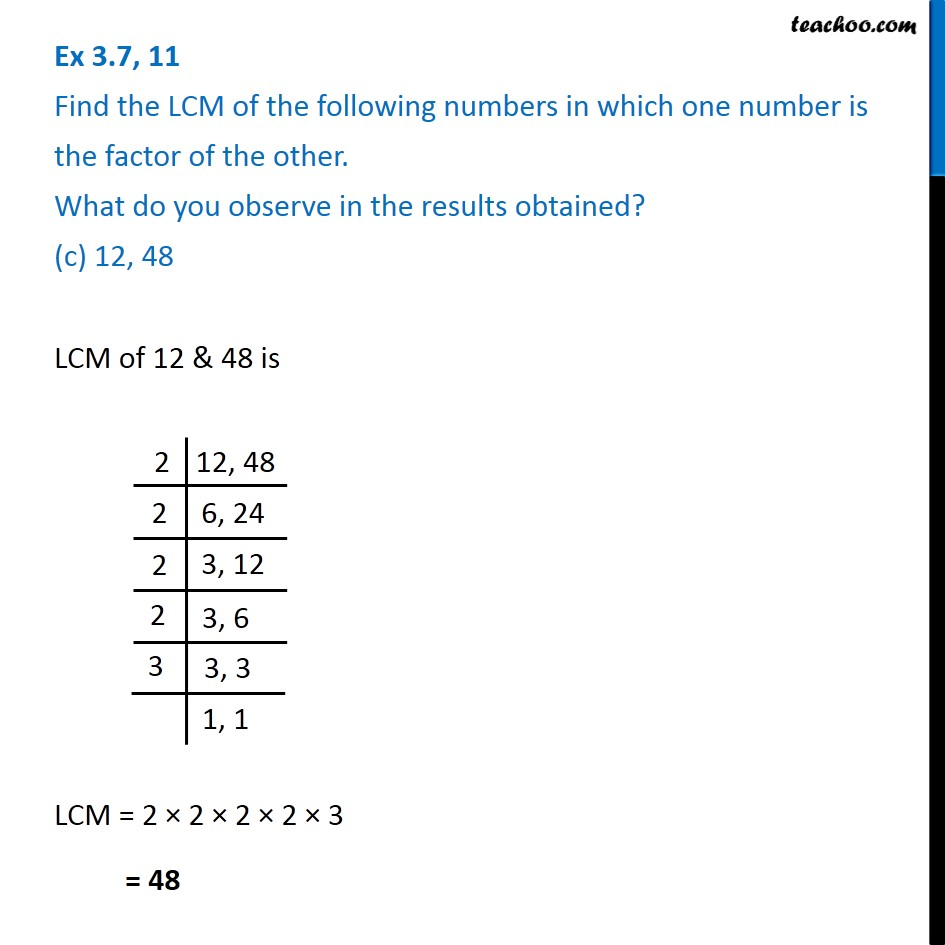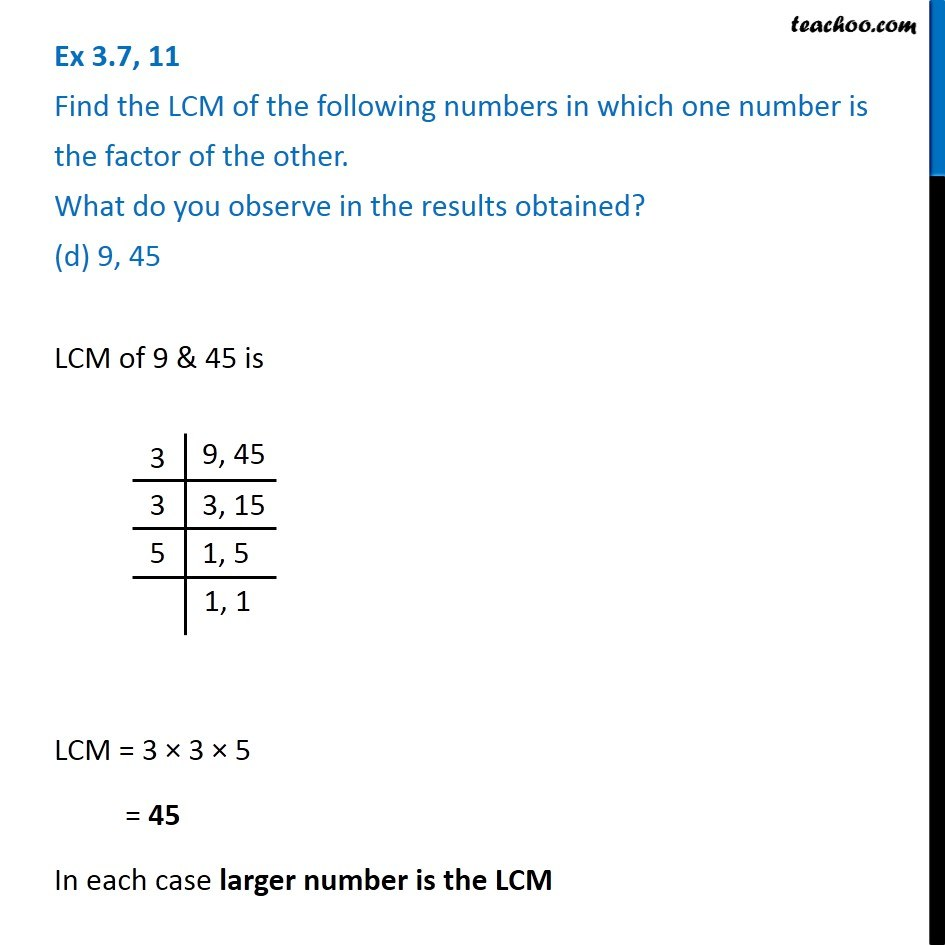1. Chapter 3 Class 6 Playing with Numbers
2. Concept wise
3. Lowest Common Multiple

Transcript

LCM of 5 & 20 Required LCM = 2 × 2 × 5 = 20 LCM of 6 & 18 is LCM = 2 × 3 × 3 = 18 LCM of 12 & 48 is LCM = 2 × 2 × 2 × 3 × 3 = 48 LCM of 9 & 45 is LCM = 3 × 3 × 5 = 45 In each case larger number is the LCM

Lowest Common Multiple